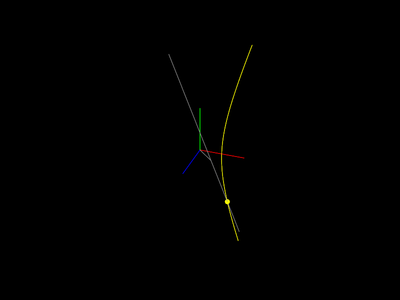Hyperbola Bar

If you take a bar and rotate it through an angle at a distance, it passes through a plane in a hyperbola. This perhaps surprising fact is demonstrated here.Ian Mallett
(geometrian)
If you take a bar and rotate it through an angle at a distance, it passes through a plane in a hyperbola. This perhaps surprising fact is demonstrated here.

Changes